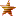###Author Topic: mc-Dev Board is now available for purchase!  (Read 146 times)

#### mc-T2

• Sr. Member
••• Posts: 252
• mc-Things! The opportunities are endless!`Define PinMode Pin2 As AnalogInput Alias lightVoltageDefine PinMode Pin3 As DigitalOutputDefine PinMode Pin4 As DigitalOutputDefine PinMode Pin5 As DigitalOutputDefine PinMode Pin6 As DigitalOutputDefine PinMode Pin7 As DigitalOutputDefine PinMode Pin8 As DigitalOutputClass ldrTest    Shared Event Boot()         Pin3 = False        Pin4 = False        Pin5 = False        Pin6 = False        Pin7 = False        Pin8 = False        LedRed = False        LedGreen = False     End Event    Shared Event measureLight() RaiseEvent Every 50 milliSeconds        Thread.Sleep(20000) 'sleep 20ms for voltage to stabilize        Dim voltage As Short = lightVoltage        If voltage > 3000 Then            Pin3 = True            Pin4 = True            Pin5 = True            Pin6 = True            Pin7 = True            Pin8 = True        ElseIf voltage <= 3000 And voltage >= 2500 Then            Pin3 = False            Pin4 = True            Pin5 = True            Pin6 = True            Pin7 = True            Pin8 = True        ElseIf voltage <= 2499 And voltage >= 2000 Then            Pin3 = False            Pin4 = False            Pin5 = True            Pin6 = True            Pin7 = True            Pin8 = True        ElseIf voltage <= 1999 And voltage >= 1500 Then            Pin3 = False            Pin4 = False            Pin5 = False            Pin6 = True            Pin7 = True            Pin8 = True        ElseIf voltage <= 1499 And voltage >= 1000 Then            Pin3 = False            Pin4 = False            Pin5 = False            Pin6 = False            Pin7 = True            Pin8 = True        ElseIf voltage < 999 And voltage >= 500 Then            Pin3 = False            Pin4 = False            Pin5 = False            Pin6 = False            Pin7 = False            Pin8 = True        ElseIf voltage < 500 Then            Pin3 = False            Pin4 = False            Pin5 = False            Pin6 = False            Pin7 = False            Pin8 = False        Else           End If    End Event    'Battery check    Shared Event measureVoltage() RaiseEvent Every 2 Hours        Dim BattVolt As Short = Device.BatteryVoltage        If BattVolt < 2200 Then            Lplan.IFTTT("mjgu7QocyzTNDUbzgitzHxBYPFaXckJrJ2LbLq8XMMg", "GarageBatt")        Else        End If    End EventEnd Class`